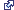# NumPy in ArcGIS

Numerical Python (NumPy) is a fundamental package for scientific computing in Python, including support for a powerful N-dimensional array object. NumPy allows you to perform complex mathematical operations. For more information, see the NumPy website.

A NumPy array is designed to work with large arrays. There are many existing Python functions that have been created to process NumPy arrays, the most noted being contained in the SciPy scientific computing package for Python.

## Tables and feature data

You can convert table and feature classes to and from NumPy arrays using functions in the data access (arcpy.da) module.

To convert NumPy arrays to tables and feature classes, the arrays must be structured arrays. Structured arrays include fields (or structs) that are used to map the data to field in ArcGIS table and feature classes. For more information on structured arrays, see Structured arrays.

Create a structured NumPy array.

``````import numpy

arr = numpy.array([(471316.383, 5000448.782), (470402.493, 5000049.216)],
numpy.dtype([('X', '>f8'),('Y', '>f8')]))``````

Once created, a structured NumPy array can be converted to a feature class or table.

Convert a NumPy array to a geodatabase feature class.

``````import arcpy
import numpy

out_fc = 'C:/data/texas.gdb/fd/pointlocations'

# Create a numpy array with an id field, and a field with a tuple
#  of x,y coordinates
arr = numpy.array([(1, (471316.3835861763, 5000448.782036674)),
(2, (470402.49348005146, 5000049.216449278))],
numpy.dtype([('idfield', numpy.int32),('XY', '<f8', 2)]))

# Define a spatial reference for the output feature class
spatial_ref = arcpy.Describe('C:/data/texas.gdb/fd').spatialReference

# Export the numpy array to a feature class using the XY field to
#  represent the output point feature
arcpy.da.NumPyArrayToFeatureClass(arr, out_fc, ['XY'], spatial_ref)``````

#### arcpy.da functions for working with tables and feature data

FunctionExplanation
ExtendTable

Join the contents of a NumPy structured array to a table based on a common attribute field.

FeatureClassToNumPyArray

Convert a feature class to a NumPy structured array.

NumPyArrayToFeatureClass

Convert a NumPy structured array to a feature class.

NumPyArrayToTable

Convert a NumPy structured array to a table.

TableToNumPyArray

Convert a table to a NumPy structured array.

Integer fields in NumPy arrays do not support nulls. If data converted using FeatureClassToNumPyArray or TableToNumPyArray contains nulls, the rows containing the nulls should either be skipped entirely or masked with a substitute value.

Skip all records that include a null.

``arr = arcpy.da.FeatureClassToNumPyArray(fc, fields, skip_nulls=True)``

Mask each None in integer fields with different values using a dictionary.

``````fields = ['field1', 'field2']
arcpy.da.FeatureClassToNumPyArray(fc, fields, null_value=-9999)``````

### Type conversions

The dtypes of the created array are determined from the field type of the input table or feature class.

Field typeNumPy dtype

Short

numpy.int32

Long

numpy.int32

Float

numpy.float32

Double

numpy.float64

Text

<u1, <u10, and so on

Date

<M8[us]

Object ID

numpy.int32

Guid

<U64

##### 注意:

String fields converted to an array will have the same width. For instance, a string field with a width of 20 will have a dtype of <u20.

Other field types not listed above, including raster and BLOB fields, are not supported. Geometry fields are also not supported, but you can add multiple geometry properties to the array using the special tokens listed below.

TokenDescription

SHAPE@XY

フィーチャの重心を表す X 座標と Y 座標の組み合わせ

SHAPE@XYZ

フィーチャの重心を表す XYZ 座標の組み合わせ

SHAPE@TRUECENTROID

フィーチャの重心を表す X 座標と Y 座標の組み合わせ SHAPE@XY と同じ値が返されます。

SHAPE@X

フィーチャの X 座標 (Double)

SHAPE@Y

フィーチャの Y 座標 (Double)

SHAPE@Z

フィーチャの Z 座標 (Double)

SHAPE@M

フィーチャの M 値 (Double)

SHAPE@JSON

ジオメトリを表す Esri JSON 文字列

SHAPE@WKB

OGC ジオメトリの WKB (Well-Known Binary) 表現。 ジオメトリ値の汎用的な表現が、連続的なバイト ストリームとして提供されます。

SHAPE@WKT

OGC ジオメトリの WKT (Well-Known Text) 表現。 ジオメトリ値の汎用的な表現が、テキスト文字列として提供されます。

SHAPE@AREA

フィーチャの面積 (Double)

SHAPE@LENGTH

フィーチャの長さ (Double)

OID@

Object ID フィールドの値

### Memory considerations

An array that requires more memory than is available will fail with a MemoryError exception.

The following are tips to avoid MemoryError exceptions:

• Delete array objects after use; deleting the array will release the memory.
• Use only those fields you need, especially text fields; a text field converted to an array will consume 4 bytes for every character of width. For instance, a string field with a width of 100 will consume 400 bytes of memory for each value in the array.
NumPy dtypeNumber of bytes per value

<U1

4

numpy.int32

4

numpy.float32

4

numpy.float64

8

##### 注意:

numpy.nbytes returns a dictionary of dtypes and number of bytes.

## Rasters

You can convert rasters to and from NumPy arrays using the ArcPy functions RasterToNumPyArray and NumPyArrayToRaster. You may want to convert an ArcGIS raster to a NumPy array to do the following:

• Implement one of the many existing Python functions that can be applied to a NumPy array (for example, run filters on the data, perform multidimensional analysis, or use optimization routines).
• Develop a custom function by accessing the individual cells within the NumPy array (for example, to implement neighborhood notation, change individual cell values, or run accumulative operators on an entire raster).

#### arcpy functions for working with rasters

FunctionExplanation
RasterToNumPyArray

Convert a raster to a NumPy array.

NumPyArrayToRaster

Convert a NumPy array to a raster.

A raster is converted to a NumPy array to calculate the percentage of the cell value in the entire raster row. A new raster is then created.

``````import arcpy
import numpy

my_array = arcpy.RasterToNumPyArray('C:/data/inRaster')
my_array_sum = my_array.sum(1)
my_array_sum.shape = (my_array.shape, 1)
my_array_perc = (my_array * 1.0) / my_array_sum

new_raster = arcpy.NumPyArrayToRaster(my_array_perc)
new_raster.save("C:/output/fgdb.gdb/PercentRaster")``````